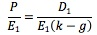### Why should I choose AnalystNotes?

AnalystNotes specializes in helping candidates pass. Period.

##### Subject 4. Multiplier Models
A price multiple is a ratio of a stock's price to a measure of value per share, such as earnings, assets, sales, or cash flows. To tie a company's performance with its market valuation, an analyst needs to evaluate what a dollar of share price can "buy" in terms of the company's earnings, sales, cash flow, or assets. For example, an analyst can estimate that each dollar invested in the company generates \$1.50 of sales. Using this information, the analyst can evaluate the attractiveness of investing in the company's stock at the current market price per share.

The P/E ratio compares stocks on the basis of how many dollars an investor is willing to pay for a dollar of expected earnings. It is also called the earnings multiplier.

The two alternative types of P/E are trailing P/E and leading P/E.

• A trailing P/E (also current P/E) is a price multiple comparing the stock's current market price to the company's earnings during the last four fiscal quarters. The EPS in such calculations are sometimes referred to as trailing twelve months (TTM) EPS. Trailing P/E is the price/earnings ratio published in stock listings of financial newspapers.

• A leading P/E (also forward P/E or prospective P/E) is a price multiple comparing the stock's current market valuation with the company's forecasted earnings for the next full fiscal year or for the next four quarters.

You can use the DDM to develop the earnings multiplier model:

• From DDM: P = D1/(k - g)
• Divide both side of the above equation by E1:For example, a stock has an expected dividend payout ratio of 90%, a required rate of return of 15%, and expected growth rate of 10%. The P/E ratio is 0.9/(0.15 - 0.1) = 18. If an investor has projected next year's earnings to be \$5, the current value of the stock is \$5 x 18 = \$90.

Thus the P/E ratio is determined by:

• The expected dividend payout ratio;
• The estimated required rate of return on the stock (k);
• The expected growth rate of dividends for the stock.

Since a firm's long-run target payout ratio is rather stable, the spread between k and g is the main determinant of the size of the P/E ratio.

A price-to-book ratio is a price multiple comparing a company's current market share price to its book value per share.

A P/S multiple is a price multiple that divides the price per share by the last 12 months' net sales per share.

A price-to-cash-flow ratio equals market price per share / cash flow per share.

The Method of Comparables

Price multiples are price-scaled by a measure of value, which provides the basis for the method of comparables. The method involves the comparison of a company's actual price multiple to some benchmark value to evaluate if an asset is relatively fairly priced, relatively undervalued, or relatively overvalued. The economic rationale is the law of one price - similar assets should sell at approximately equal prices. That is, if two companies are identical in all respects, their shares should be quoted at the same price in an efficient market.

Some of the most widely used benchmarks involve the multiple of a closely matched individual stock and the average or median value of the multiple for the stock's company or industry peer group.

This method is the most popular application of price multiples. It allows investors to determine a stock's relative valuation as compared to the benchmark. For example, many analysts point out that even after the technology market crash of 2000-2001, some technology stocks still remain overvalued on the basis of the P/E multiple compared to normal historical valuations.

By its nature, the method of comparables allows analysts to value investments only on a relative basis (i.e., only in comparison to the benchmark value of multiples). It cannot be used for absolute valuation of stocks, since the benchmark itself may well depart from its fair value. Although Sun Microsystems seems to be undervalued compared to other storage hardware companies, an analyst would need more information to recommend purchase of its stocks, since the entire storage industry may currently be overvalued and primed for decline.

Learning Outcome Statements

g. explain the rationale for using price multiples to value equity, how the price to earnings multiple relates to fundamentals, and the use of multiples based on comparables;

h. calculate and interpret the following multiples: price to earnings, price to an estimate of operating cash flow, price to sales, and price to book value;

CFA® 2021 Level I Curriculum, , Volume 5, Reading 41